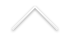The main objective of Dynamical System Theory is to understand the asymptotic behavior of a system that is given by a transformation or a differential equation as well as its orbits. The orbit describes the movement of a point (initial condition) under the action of the transformation or flow.

One central problem is to understand the qualitative behavior, understand when the behavior remains unchanged under small perturbations, and when there appear qualitative changed (bifurcations).

A dynamical system is said to be chaotic if it shows sensitive dependence on the initial conditions that typically results in the inpredictability of the asymptotic behavior of individual orbits. In particular, such behavior creates problems in computer simulations. The theory of uniformly hyperbolic dynamical systems forms an important sub-area in the qualitative studies of chaotic dynamics. However, this theory does not cover many interesting systems such as the Lorenz system and the Henon system, which contributed significantly to the investigation of non-uniformly hyperbolic dynamical systems. In fact, dynamical systems that fell out of concrete problems in physics rarely are uniformly hyperbolic. This indicates the necessity of understanding the mathematical foundations of the theory of hyperbolic dynamics in a more general context. Moreover, it is important to understand typical phenomena that appear with non-uniformly hyperbolic dynamical systems.

Main Research Areas of the Group Include:

• (non-uniformly, partially hyperbolic etc.) dynamical systems
• ergodic theory
• bifurcation theory of chaotic attractors
• connections with differential geometry

Researchers:

• Alexander Arbieto
• Katrin Gelfert
• Maria Jose Pacifico
• Samuel Senti
• Nuno Luzia
• Carlos Morales